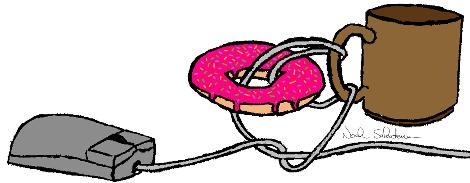Research InterestsMy main interests are quantum topology, mathematical models of topological phases of matter, and their application to quantum physics and quantum computing. I am currently working on the mathematical foundations of topological quantum computation, mainly topological quantum field theory and higher algebroid theory. I consider this research as part of quantum mathematics—a catch-call for mathematics needed to model quantum systems and mathematics based on quantum logic.
« Return
 Curriculum Vitae Perimeter Institute BiCMR Personal